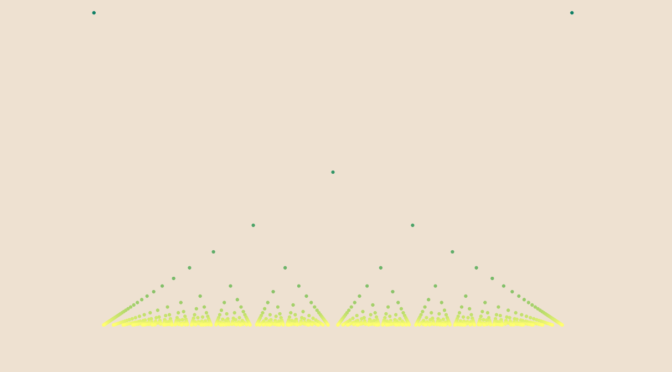# A semi-continuous function with a dense set of points of discontinuity

Let’s come back to Thomae’s function which is defined as:
$f: \left|\begin{array}{lrl} \mathbb{R} & \longrightarrow & \mathbb{R} \\ x & \longmapsto & 0 \text{ if } x \in \mathbb{R} \setminus \mathbb{Q} \\ \frac{p}{q} & \longmapsto & \frac{1}{q} \text{ if } \frac{p}{q} \text{ in lowest terms and } q > 0 \end{array}\right.$

We proved here that $$f$$ right-sided and left-sided limits vanish at all points. Therefore $$\limsup\limits_{x \to a} f(x) \le f(a)$$ at every point $$a$$ which proves that $$f$$ is upper semi-continuous on $$\mathbb R$$. However $$f$$ is continuous at all $$a \in \mathbb R \setminus \mathbb Q$$ and discontinuous at all $$a \in \mathbb Q$$.